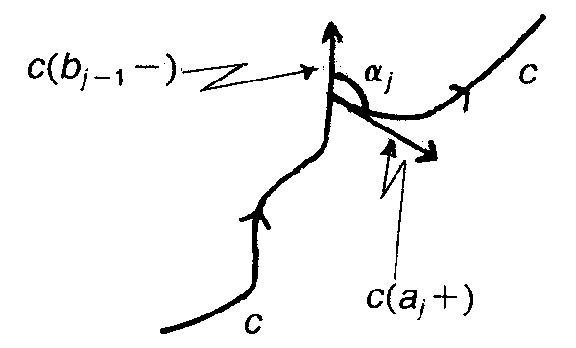# Difference between revisions of "Rotation number"

Let $c: [ a, b] \rightarrow \mathbf R ^ {2}$ be a regular curve, i.e. $c( t)$ is smooth and $\dot{c} ( t) \neq 0$ for all $t \in [ a, b]$. Then there is a continuous piecewise-differentiable function $\theta ( t)$ such that $\dot{c} ( t) / | \dot{c} ( t) |$, the normalized velocity vector at $c( t)$, is equal to $( \cos \theta ( t), \sin \theta ( t))$. Moreover, the difference $\theta ( b) - \theta ( a)$ is independent of the choice of $\theta$.Figure: r082650a

Now, let $c: [ 0, A] \rightarrow \mathbf R ^ {2}$ be a piecewise-smooth regular closed curve and let $0= b _ {-} 1 = a _ {0} < b _ {0} = a _ {1} < \dots < b _ {k} = A$ partition $[ 0, A]$ into intervals such that $c$ restricted to $[ a _ {j} , b _ {j} ]$ is differentiable for all $j$. Let $\alpha _ {j}$ be the exterior angle between the tangent vectors at the corner at $c( b _ {j-} 1 ) = c( a _ {j} )$, i.e. $\alpha _ {j}$ is the angle between $\dot{c} ( b _ {j-} 1 - )$ and $\dot{c} ( a _ {j} +)$( with $- \pi < \alpha _ {j} \leq \pi$). The number

$$n _ {c} = \frac{1}{2 \pi } \sum _ { j } ( \theta _ {j} ( b _ {j} ) - \theta _ {j} ( a _ {j} )) + \frac{1}{2 \pi } \sum _ { j } \alpha _ {j}$$

is called the rotation number of the curve $c$.

If $\mathbf R ^ {2}$ is identified with the complex plane $\mathbf C$ and $c$ is smooth (so that all $\alpha _ {j}$ are zero), then $n _ {c}$ is the winding number of the closed curve $t \mapsto \dot{c} ( t) / | \dot{c} ( t) |$ with respect to the origin.

Let $c: [ 0, A] \rightarrow \mathbf R ^ {2}$ be piecewise-smooth, regular, closed, and simple (i.e. no self-intersections), and suppose that the exterior angles are always $\neq \pi$ in absolute value. Then the so-called Umlaufsatz says that $n _ {c} = \pm 1$, depending on the orientation. From this it is easy to calculate the $n _ {c}$ of closed curves with self-intersections. For instance, the rotation number of the figure eight curve is zero.

It readily follows from these results that, e.g., the sum of the interior angles of a convex $n$- gon is $( n- 2) \pi$. There also result the various formulas for triangles (and other figures) made up of circle segments, such as $\alpha _ {1} + \alpha _ {2} + \alpha _ {3} + \beta _ {1} + \beta _ {2} + \beta _ {3} = 180 \circ$ in the case of the circle segment triangle depicted on the left in Fig.a2; and $- \alpha _ {1} + \alpha _ {2} + \alpha _ {3} - \beta _ {1} + \beta _ {2} + \beta _ {3} = 180 \circ$ for the circle segment triangle depicted on the right in Fig.a2. Here the $\beta _ {i}$ denote the number of degrees of the circle segments in question, $0 \leq \beta _ {i} \leq 360 \circ$, $i= 1, 2, 3$.Figure: r082650b

For more on the planar geometry of circle segment triangles and such, see, e.g., [a2], [a3].

How to Cite This Entry:
Rotation number. Encyclopedia of Mathematics. URL: http://encyclopediaofmath.org/index.php?title=Rotation_number&oldid=12710